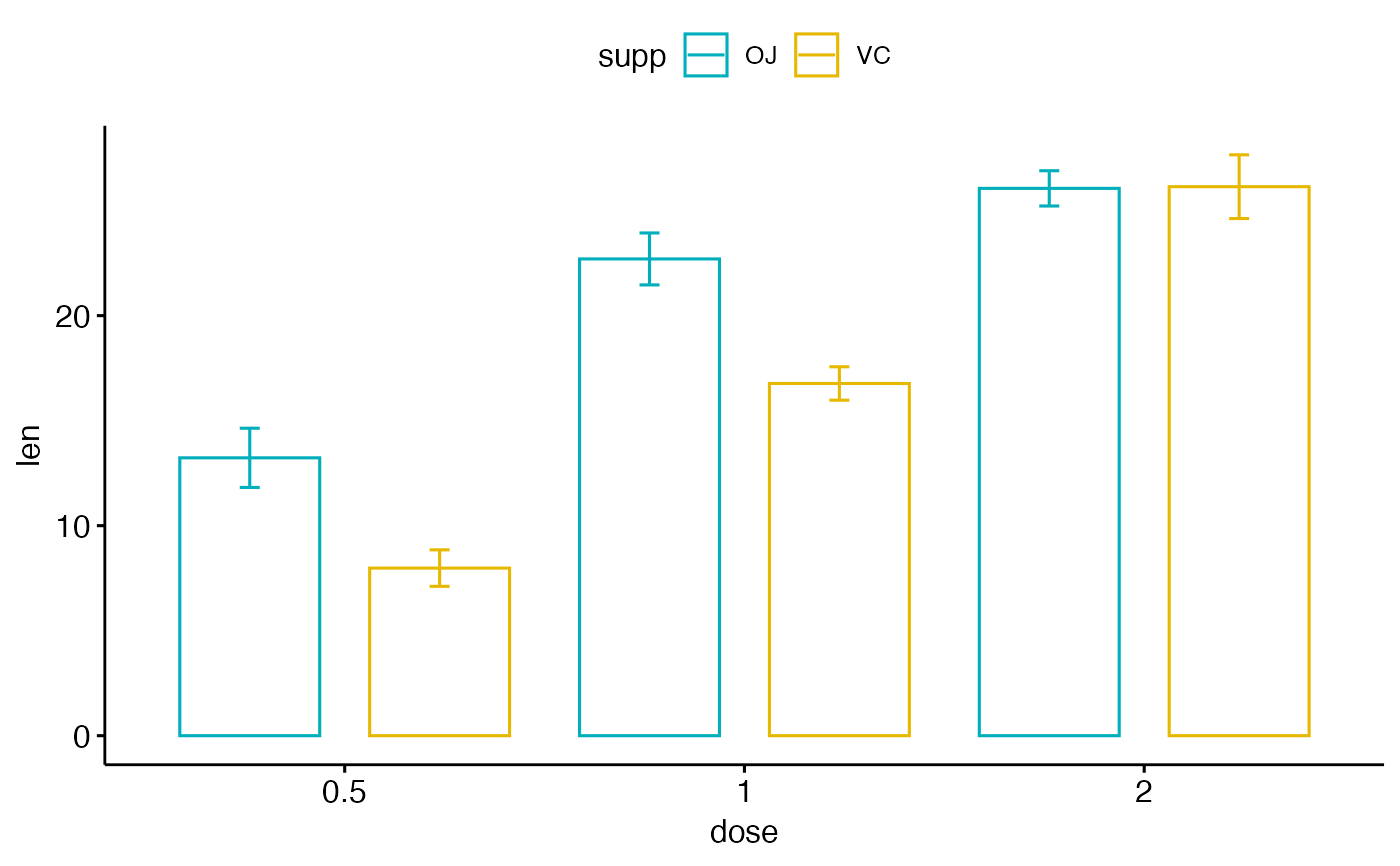Create a bar plot.

ggbarplot(
data,
x,
y,
combine = FALSE,
merge = FALSE,
color = "black",
fill = "white",
palette = NULL,
size = NULL,
width = NULL,
title = NULL,
xlab = NULL,
ylab = NULL,
facet.by = NULL,
panel.labs = NULL,
short.panel.labs = TRUE,
select = NULL,
remove = NULL,
order = NULL,
error.plot = "errorbar",
label = FALSE,
lab.col = "black",
lab.size = 4,
lab.pos = c("out", "in"),
lab.vjust = NULL,
lab.hjust = NULL,
lab.nb.digits = NULL,
sort.val = c("none", "desc", "asc"),
sort.by.groups = TRUE,
top = Inf,
position = position_stack(),
ggtheme = theme_pubr(),
...
)

## Arguments

data a data frame x and y variables for drawing. logical value. Default is FALSE. Used only when y is a vector containing multiple variables to plot. If TRUE, create a multi-panel plot by combining the plot of y variables. logical or character value. Default is FALSE. Used only when y is a vector containing multiple variables to plot. If TRUE, merge multiple y variables in the same plotting area. Allowed values include also "asis" (TRUE) and "flip". If merge = "flip", then y variables are used as x tick labels and the x variable is used as grouping variable. outline and fill colors. the color palette to be used for coloring or filling by groups. Allowed values include "grey" for grey color palettes; brewer palettes e.g. "RdBu", "Blues", ...; or custom color palette e.g. c("blue", "red"); and scientific journal palettes from ggsci R package, e.g.: "npg", "aaas", "lancet", "jco", "ucscgb", "uchicago", "simpsons" and "rickandmorty". Numeric value (e.g.: size = 1). change the size of points and outlines. numeric value between 0 and 1 specifying box width. plot main title. character vector specifying x axis labels. Use xlab = FALSE to hide xlab. character vector specifying y axis labels. Use ylab = FALSE to hide ylab. character vector, of length 1 or 2, specifying grouping variables for faceting the plot into multiple panels. Should be in the data. a list of one or two character vectors to modify facet panel labels. For example, panel.labs = list(sex = c("Male", "Female")) specifies the labels for the "sex" variable. For two grouping variables, you can use for example panel.labs = list(sex = c("Male", "Female"), rx = c("Obs", "Lev", "Lev2") ). logical value. Default is TRUE. If TRUE, create short labels for panels by omitting variable names; in other words panels will be labelled only by variable grouping levels. character vector specifying which items to display. character vector specifying which items to remove from the plot. character vector specifying the order of items. character vector for adding another plot element (e.g.: dot plot or error bars). Allowed values are one or the combination of: "none", "dotplot", "jitter", "boxplot", "point", "mean", "mean_se", "mean_sd", "mean_ci", "mean_range", "median", "median_iqr", "median_hilow", "median_q1q3", "median_mad", "median_range"; see ?desc_statby for more details. parameters (color, shape, size, fill, linetype) for the argument 'add'; e.g.: add.params = list(color = "red"). plot type used to visualize error. Allowed values are one of c("pointrange", "linerange", "crossbar", "errorbar", "upper_errorbar", "lower_errorbar", "upper_pointrange", "lower_pointrange", "upper_linerange", "lower_linerange"). Default value is "pointrange" or "errorbar". Used only when add != "none" and add contains one "mean_*" or "med_*" where "*" = sd, se, .... specify whether to add labels on the bar plot. Allowed values are: logical value: If TRUE, y values is added as labels on the bar plot character vector: Used as text labels; must be the same length as y. text color and size for labels. character specifying the position for labels. Allowed values are "out" (for outside) or "in" (for inside). Ignored when lab.vjust != NULL. numeric, vertical justification of labels. Provide negative value (e.g.: -0.4) to put labels outside the bars or positive value to put labels inside (e.g.: 2). numeric, horizontal justification of labels. integer indicating the number of decimal places (round) to be used. a string specifying whether the value should be sorted. Allowed values are "none" (no sorting), "asc" (for ascending) or "desc" (for descending). logical value. If TRUE the data are sorted by groups. Used only when sort.val != "none". a numeric value specifying the number of top elements to be shown. Position adjustment, either as a string, or the result of a call to a position adjustment function. function, ggplot2 theme name. Default value is theme_pubr(). Allowed values include ggplot2 official themes: theme_gray(), theme_bw(), theme_minimal(), theme_classic(), theme_void(), .... other arguments to be passed to be passed to ggpar().

## Details

The plot can be easily customized using the function ggpar(). Read ?ggpar for changing:

• main title and axis labels: main, xlab, ylab

• axis limits: xlim, ylim (e.g.: ylim = c(0, 30))

• axis scales: xscale, yscale (e.g.: yscale = "log2")

• color palettes: palette = "Dark2" or palette = c("gray", "blue", "red")

• legend title, labels and position: legend = "right"

• plot orientation : orientation = c("vertical", "horizontal", "reverse")

ggpar, ggline

## Examples

# Data
df <- data.frame(dose=c("D0.5", "D1", "D2"),
len=c(4.2, 10, 29.5))
print(df)#>   dose  len
#> 1 D0.5  4.2
#> 2   D1 10.0
#> 3   D2 29.5
# Basic plot with label outsite
# +++++++++++++++++++++++++++
ggbarplot(df, x = "dose", y = "len",
label = TRUE, label.pos = "out")# Change width
ggbarplot(df, x = "dose", y = "len", width = 0.5)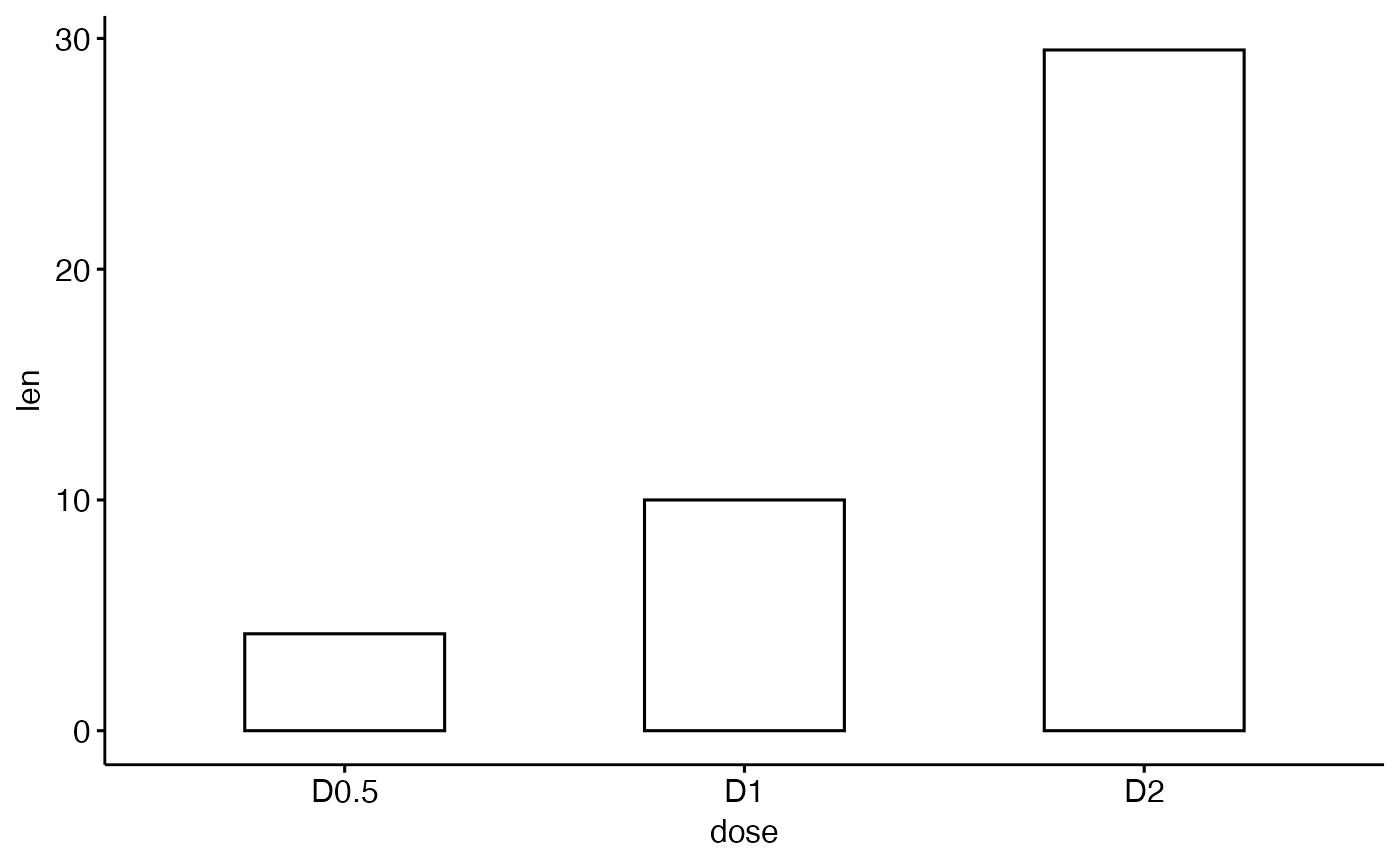# Change the plot orientation: horizontal
ggbarplot(df, "dose", "len", orientation = "horiz")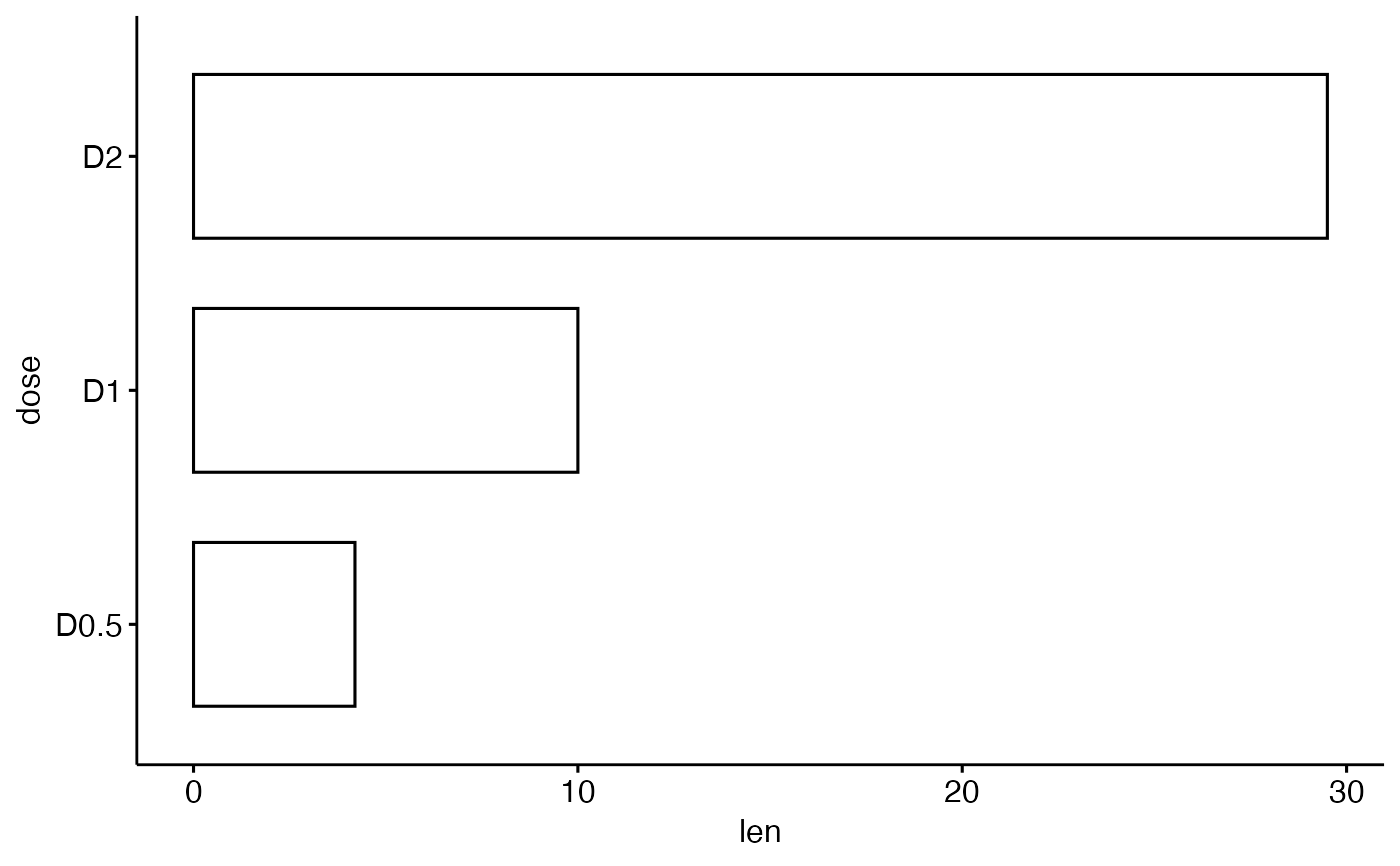# Change the default order of items
ggbarplot(df, "dose", "len",
order = c("D2", "D1", "D0.5"))# Change colors
# +++++++++++++++++++++++++++

# Change fill and outline color
ggbarplot(df, "dose", "len",
fill = "steelblue", color = "steelblue",
label = TRUE, lab.pos = "in", lab.col = "white")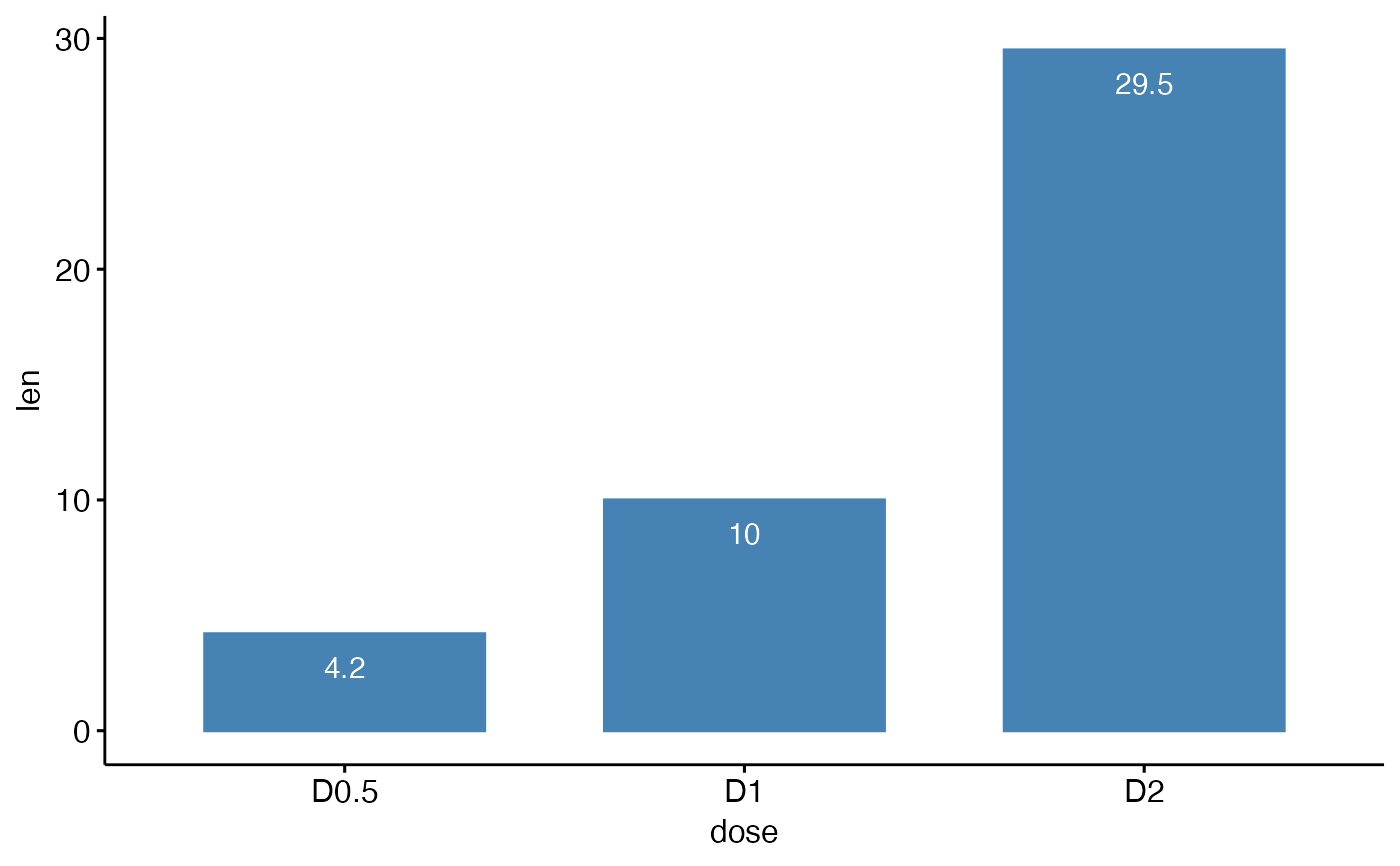# Change colors by groups: dose
# Use custom color palette
ggbarplot(df, "dose", "len", color = "dose",
palette = c("#00AFBB", "#E7B800", "#FC4E07"))# Change fill and outline colors by groups
ggbarplot(df, "dose", "len",
fill = "dose", color = "dose",
palette = c("#00AFBB", "#E7B800", "#FC4E07"))# Plot with multiple groups
# +++++++++++++++++++++

# Create some data
df2 <- data.frame(supp=rep(c("VC", "OJ"), each=3),
dose=rep(c("D0.5", "D1", "D2"),2),
len=c(6.8, 15, 33, 4.2, 10, 29.5))
print(df2)#>   supp dose  len
#> 1   VC D0.5  6.8
#> 2   VC   D1 15.0
#> 3   VC   D2 33.0
#> 4   OJ D0.5  4.2
#> 5   OJ   D1 10.0
#> 6   OJ   D2 29.5
# Plot "len" by "dose" and change color by a second group: "supp"
ggbarplot(df2, "dose", "len",
fill = "supp", color = "supp", palette = "Paired",
label = TRUE, lab.col = "white", lab.pos = "in")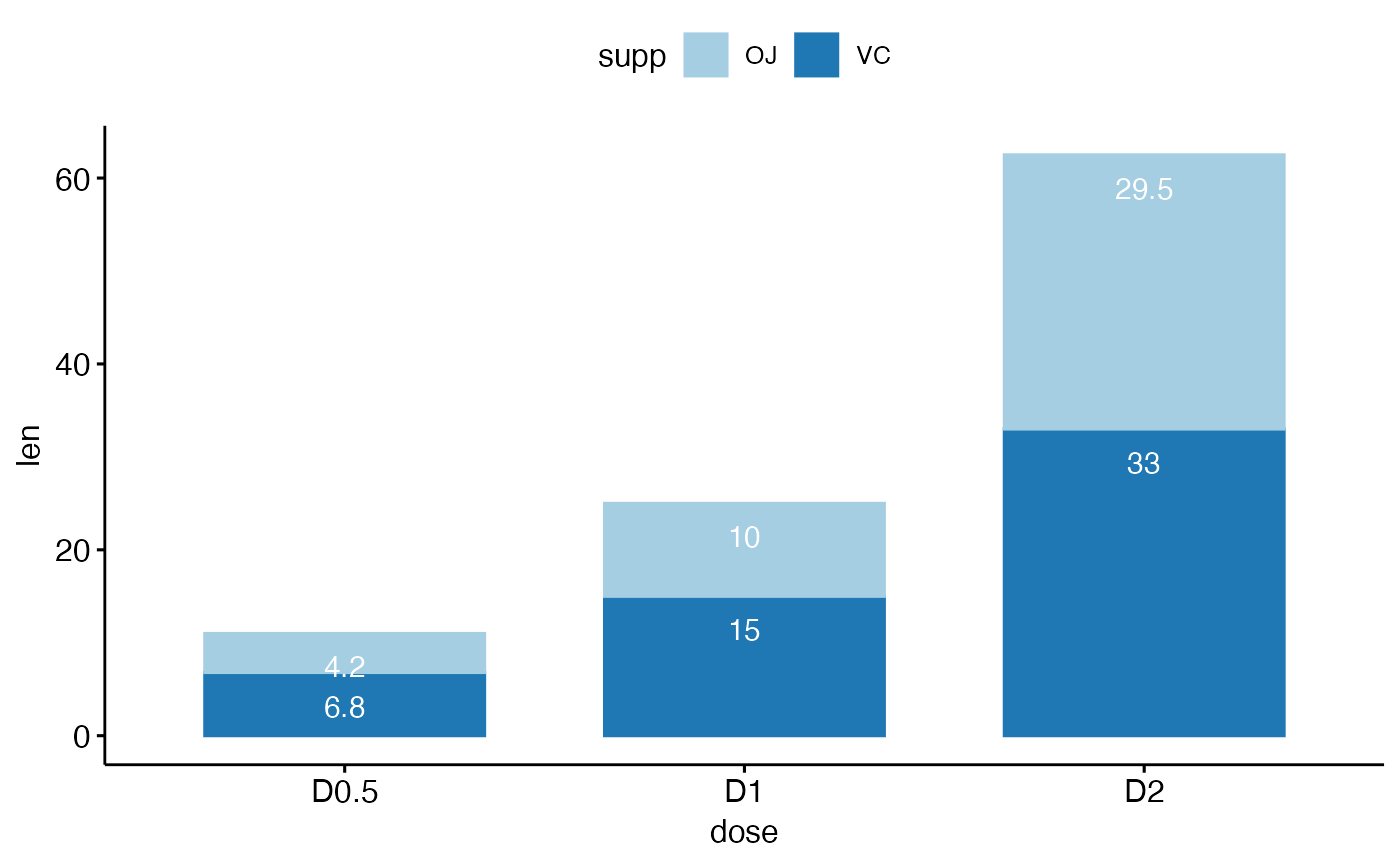# Change position: Interleaved (dodged) bar plot
ggbarplot(df2, "dose", "len",
fill = "supp", color = "supp", palette = "Paired",
label = TRUE,
position = position_dodge(0.9))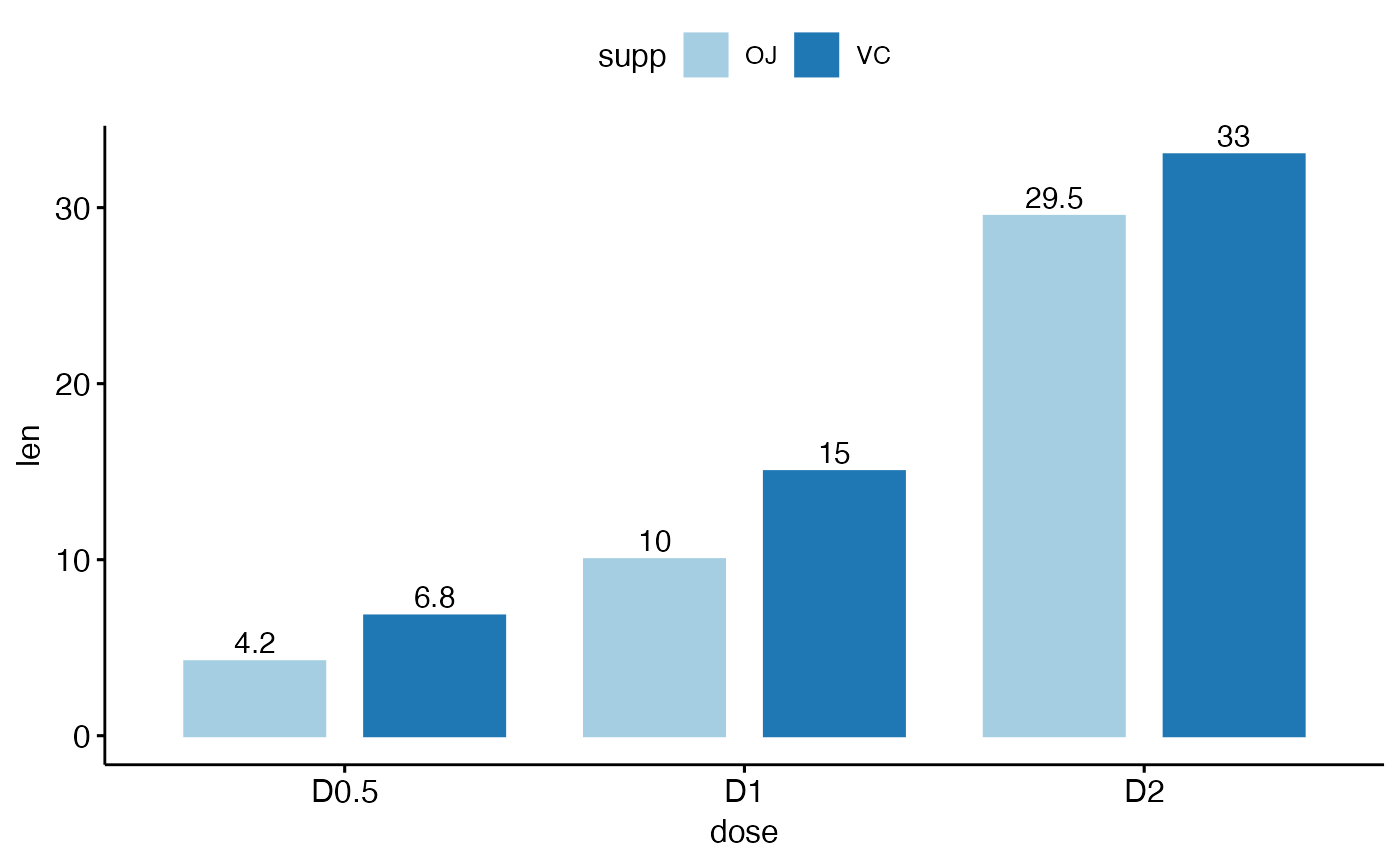# ++++++++++++++++++++++++++

# Data: ToothGrowth data set we'll be used.
df3 <- ToothGrowth
#> 1   4.2   VC  0.5
#> 2  11.5   VC  0.5
#> 3   7.3   VC  0.5
#> 4   5.8   VC  0.5
#> 5   6.4   VC  0.5
#> 6  10.0   VC  0.5
#> 7  11.2   VC  0.5
#> 8  11.2   VC  0.5
#> 9   5.2   VC  0.5
#> 10  7.0   VC  0.5
# It can be seen that for each group we have
# different values
ggbarplot(df3, x = "dose", y = "len")# Visualize the mean of each group
ggbarplot(df3, x = "dose", y = "len",
add = "mean")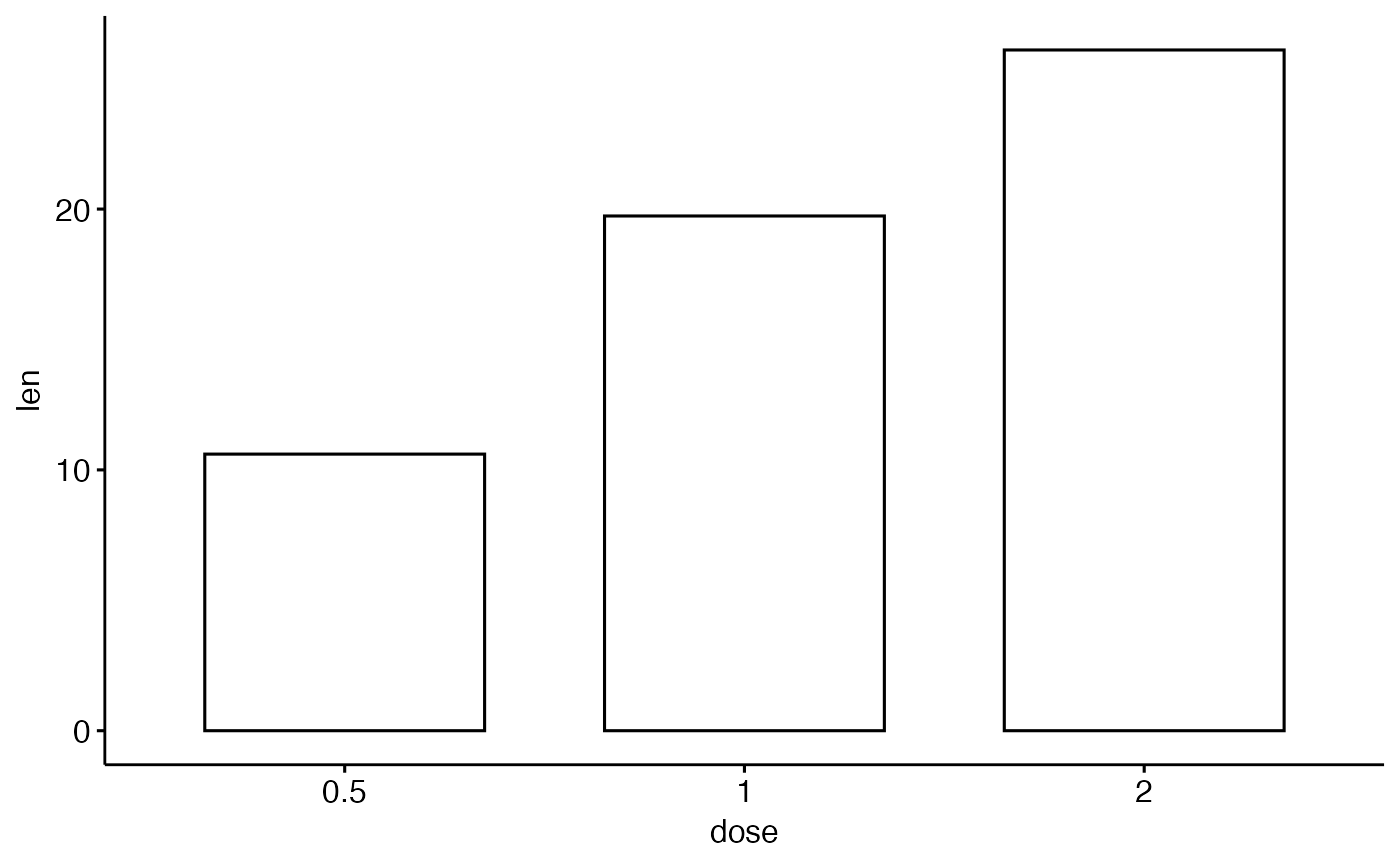# (other values include: mean_sd, mean_ci, median_iqr, ....)
ggbarplot(df3, x = "dose", y = "len",
add = "mean_se", label = TRUE, lab.vjust = -1.6)# Use only "upper_errorbar"
ggbarplot(df3, x = "dose", y = "len",
add = "mean_se", error.plot = "upper_errorbar")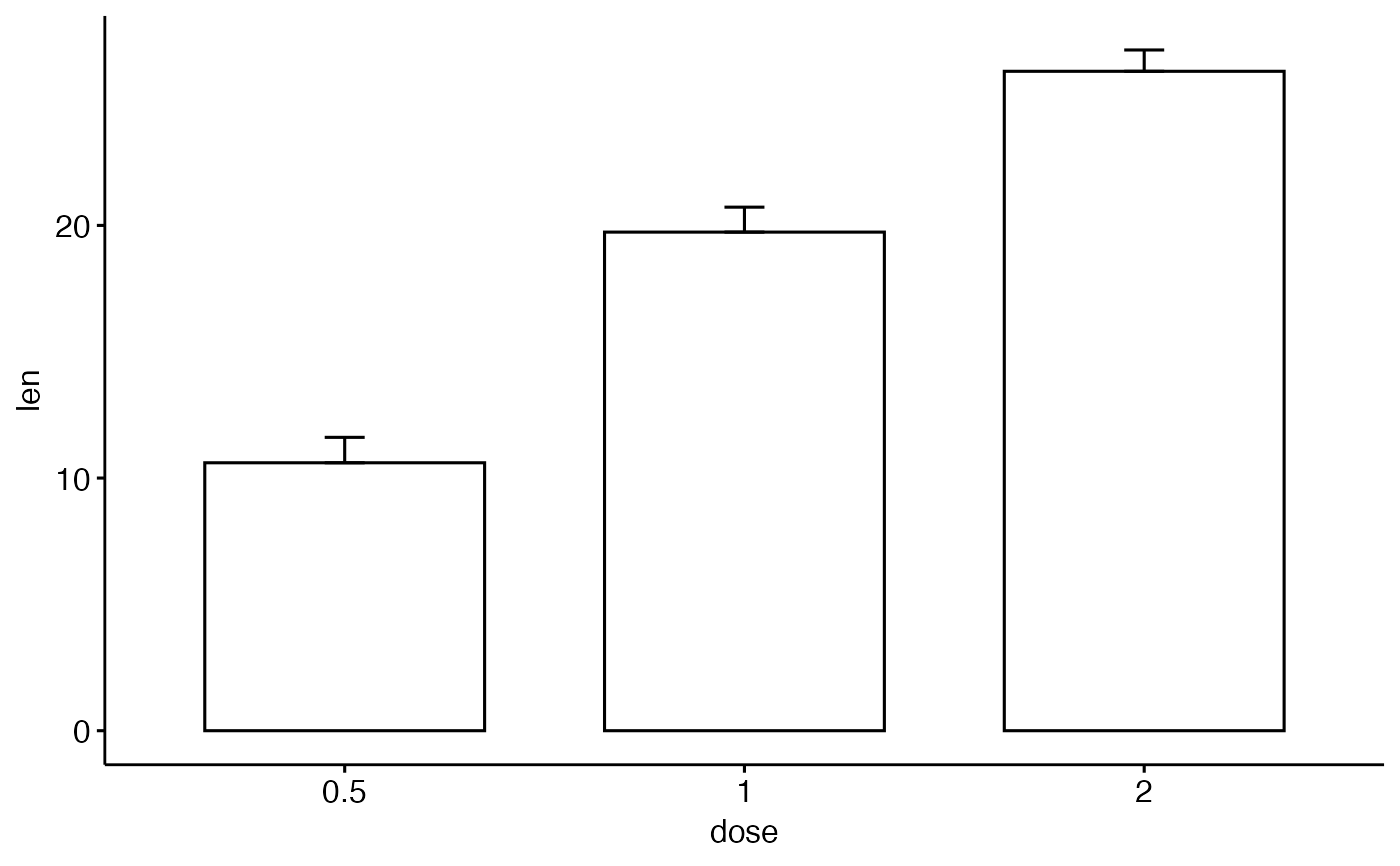# Change error.plot to "pointrange"
ggbarplot(df3, x = "dose", y = "len",
add = "mean_se", error.plot = "pointrange")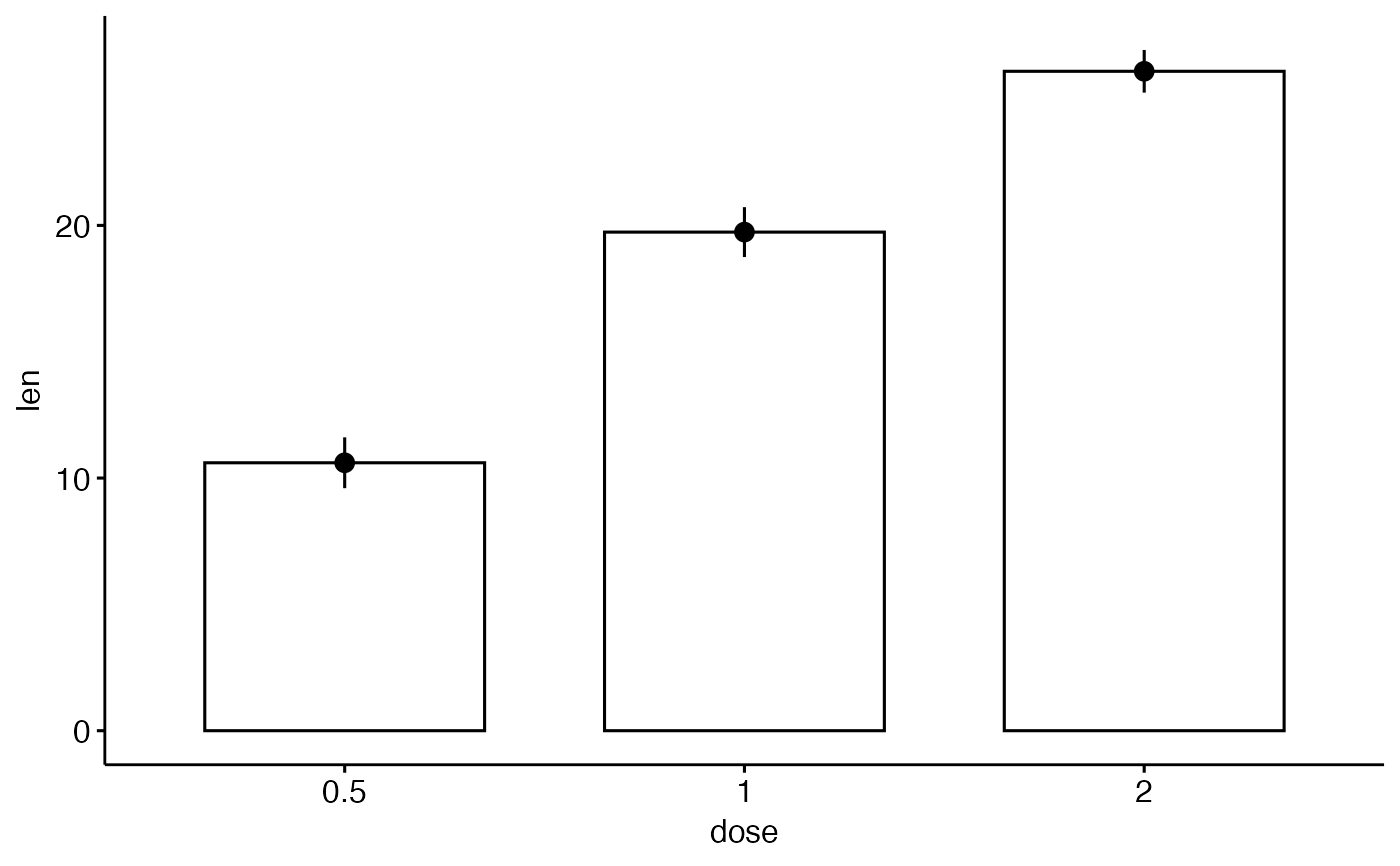# Add jitter points and errors (mean_se)
ggbarplot(df3, x = "dose", y = "len",
add = c("mean_se", "jitter"))# Add dot and errors (mean_se)
ggbarplot(df3, x = "dose", y = "len",
add = c("mean_se", "dotplot"))#> stat_bindot() using bins = 30. Pick better value with binwidth.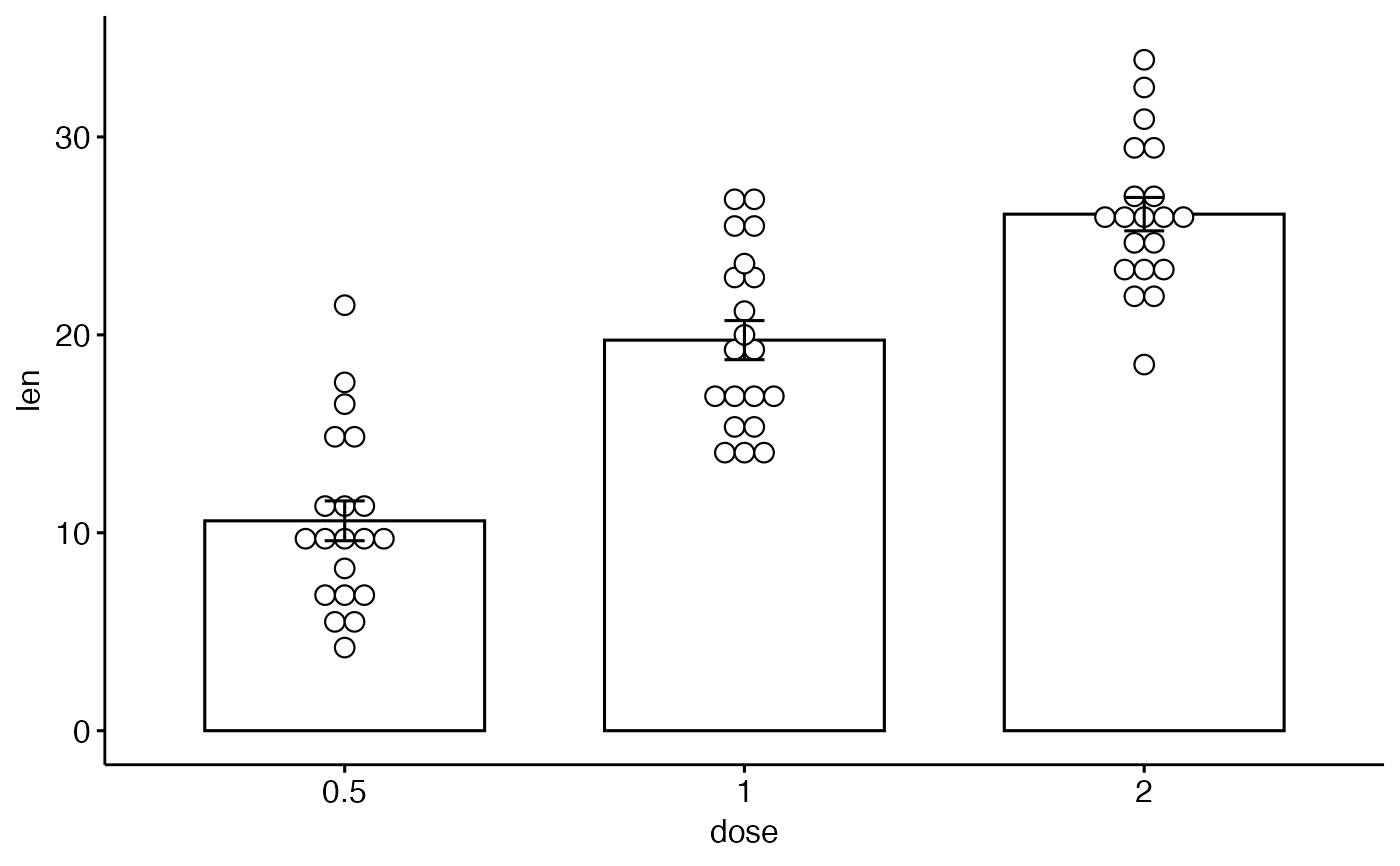# Multiple groups with error bars and jitter point
ggbarplot(df3, x = "dose", y = "len", color = "supp",
add = "mean_se", palette = c("#00AFBB", "#E7B800"),
position = position_dodge())